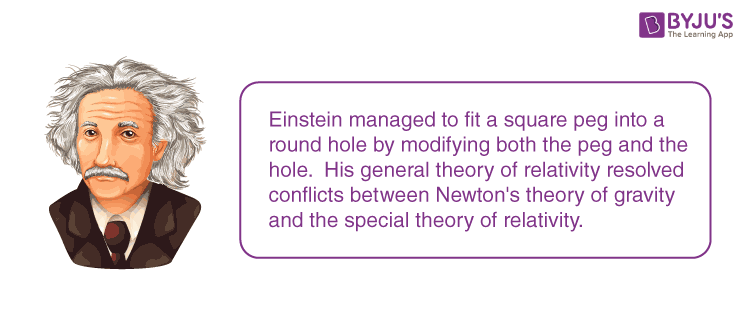# Equivalence Principle

In the general theory of relativity developed by Albert Einstein, the equivalence principle explains about “equivalence of inertial mass and gravitational mass”.

He observed that the force due to gravity (gravitational force) experienced by a person standing on a massive object (for example earth) is equivalent to the pseudo-force experienced by the observer in the non-inertial frame of reference (accelerated).

## What is Equivalence Principle?

In the theory of general relativity, the equivalence principle is the equivalence of gravitational and inertial mass, and Albert Einstein’s observation that the gravitational “force” is experienced locally while standing on a massive body (such as the Earth) is the same as the pseudo-force experienced by an observer in a non-inertial (accelerated) frame of reference.

## History of Equivalence Principle

Albert Einstein realized some complexity between Newton’s theory of gravity and his theory of special relativity. He looks into the universe in a completely different way!

As we know, in 1905, Albert Einstein set a milestone in physics with his new framework of laws of physics, which is his theory of special relativity. Nonetheless, one facet of physics seemed incomplete: Newton’s gravitational force theory. The special theory of relativity enfolded space and time excluding gravity. Years later, Albert Einstein managed to unify gravity with his relativistic ideas on space and time. As a result, another revolutionary theory emerged. “The general theory of relativity”.The very first step towards a general theory of relativity was the realization that, Even within a gravitational field, there exists a reference frame in which gravity is almost zero/absent.

Thus, at least to a certain approximation, gravity-free laws of special relativity govern the laws of physics if and only if the observations are confined within a small region of space and time. This derived from what he formulated as equivalence principle , which, in turn, is inspired by the consequence of a freefall.

## What is Effective Mass?

Mass is a property of any physical object; It is a measure of resistance to the acceleration in the presence of external force. Effective mass is the terminology used in the general theory of relativity, which talks about gravitational and inertial mass.

Gravity: Tendency of massive object to attract each other. Gravitational force between two bodies is given by equation (1):

 $$\begin{array}{l}F=G\frac{Mm_{gravity}}{r^{2}}\end{array}$$

Where,

• G is the universal gravitational constant
• M is the mass of one body
• mgravity is the mass of the second body
• r is the distance between them

Inertia: Resistance offered by the body against the change in its current state of motion. Force involved here is given by equation (2):

 $$\begin{array}{l}F=m_{inertia}\times a\end{array}$$

Where,

• minertia is the inertial mass of the object
• a is the acceleration of the object

Principle of equivalence comes up with an idea, saying equation (1) and (2) are the same. That is:

$$\begin{array}{l}F= G\frac{Mm_{gravity}}{r^{2}}=m_{inertia}\times a\end{array}$$
$$\begin{array}{l}\Rightarrow G\frac{Mm_{gravity}}{r^{2}}=m_{inertia}\times a\end{array}$$

On rearranging the above equation we get:

 $$\begin{array}{l}\Rightarrow a=\left [ \frac{m_{gravity}}{m_{inertia}} \right ]\times G\frac{M}{r^{2}}\end{array}$$

Hence, mathematical equivalence is proved.

You may also want to check out these topics given below!

## Example of Equivalence PrincipleDropping an object from an elevator cabin completely isolated from the outer world

Imagine you are standing in an elevator or, precisely, is something inside which looks very similar to an elevator cabin. You are isolated from the outer world. Under these circumstances, if you take an object and drop it, it falls and reaches the floor/base. This is the most expected way you expect, considering your experience here on earth. The situation is depicted in the diagram.

Will the effect be the same if the elevator is subjected to a gravitational field similar to the earth?Inside a rocket accelerating at 9.8 meters per square second

Not necessarily! Theoretically, you may be situated in deep space, far away from the gravitational influence of any significant mass concentration. The situation is illustrated clearly in the diagram. The cabin you are standing in can be inside a rocket accelerating at 9.8 meters per square second.

In such a scenario, you drop off an object; The object would experience an acceleration of 9.8 meters per square second from the cabin floor- the same acceleration that an object experiences during free fall here, right on earth. As an observer standing inside a cabin floor, you can hardly differentiate between two situations. From your frame of reference, situations will be indistinguishable: are they falling under the gravity of a massive object like earth? Or is it the cabin floor accelerating them?

Thus, Einstein formulates his Principle of equivalence stating that “No experiment can be performed that could distinguish between a uniform gravitational field and an equivalent uniform acceleration.”

## Watch the video to understand the history of gravitation!Stay tuned to BYJU’S and Fall in Love with Learning!

## Frequently Asked Questions – FAQs

Q1

### What are the main two theories of Albert Einstein?

The special theory of relativity and the general theory of relativity are the two important theories developed by Albert Einstein.
Q2

### What is the equivalence principle?

The equivalence principle is the fundamental law in modern physics, which describes that inertial and gravitational forces are similar in nature and usually indistinguishable.
Q3

### Who developed the equivalence principle?

Albert Einstein formulated the equivalence principle.
Q4

### What is the significance of the equation E = mc2?

The equation E = mc2 shows that energy and mass are the same entity and can be transformed into each other under ideal conditions.
Q5

### When did Albert Einstein publish the special theory of relativity?

In 1905 Albert Einstein published the special theory of relativity.
Test Your Knowledge On Equivalence Principle!